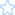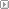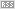####ASP代码加密| |[2006/09/23 22:20 | by ipaddr ]
ASP代码加密

ASP代码一般是明文，很少有加密的，MS有个工具Script Encoder可以加密，这个东东可以微软官方网站免费下载，而且还有详细使用说明，但是经过它加密后的文件会有<%@ language = vbscript.encode %>，管理员看到这句话就知道这个asp文件被加密了。而且也有相关的解密文件。

function UnEncode(temp)
but=1
for i =1 to len(temp)
if mid(temp,i,1)<>"汤" then
pk=asc(mid(temp,i,1))-but
if pk>126 then
pk=pk-95
elseif pk<32 then
pk=pk+95
end if
a=a&chr(pk)
else
a=a&vbcrlf
end if
next
UnEncode=a
end function

function Encode(temp)
but=1
cc=replace(temp,vbcrlf,"汤")
for i= 1 to len(cc)
if mid(cc,i,1)<>"汤" then
pk=asc(mid(cc,i,1))+but
if pk>126 then
pk=pk-95
elseif pk<32 then
pk=pk+95
end if
a=a&chr(pk)
else
a=a&"汤"
end if
next
'a=replace(a,"""","""""")
Encode=a
end function

Program | 评论(0) | 引用(24) | 阅读(4321)